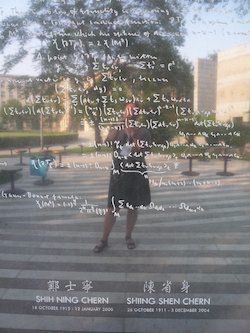# Automorphic forms and trace formulae

D. I. Wallace, Selberg's Trace Formula and units in higher degree number fields, Ph.D. Thesis, U.C.S.D., 1982.

D. I. Wallace, Conjugacy classes of hyperbolic matrices and ideal classes in an order, Trans. Amer. Math. Soc. 283 (1984), 177-184.

D. I. Wallace, Explicit form of the hyperbolic term in the Selberg Trace Formula for SL(3, Z), J. Number Theory, 24 (1986), 127-133.

D. I. Wallace, A preliminary version of the Selberg Trace Formula for SL(3,Z)\SL(3, R)/SO(3, R), Contemporary Mathematics, 53 (1986), 11-15.

D. I. Wallace, Maximal Parabolic Terms in the Selberg Trace Formula for SL(3,Z)\SL(3, R)/SO(3, R), J. Number Theory 29, (1988), 101-117.

D. I. Wallace, Minimal parabolic terms in the Selberg Trace Formula for SL(3,Z)\SL(3, R)/SO(3, R), J. Number Theory 32, (1989)

D. I. Wallace, Terms in the Selberg Trace Formula for SL(3,Z)\SL(3, R)/SO(3, R) associated to Eisenstein Series coming from a maximal parabolic subgroup, Proc. Amer. Math. Soc. 106, (1989), 875-883

D. I. Wallace, Terms in the Selberg Trace Formula for SL(3,Z)\SL(3, R)/SO(3, R) associated to Eisenstein Series coming from a minimal parabolic subgroup, Trans. Amer. Math. Soc. 327, (1991), 781-793

D. I. Wallace, The loxodromic term of the Selberg trace formula for SL(3,Z)\SL(3, R)/SO(3, R), Proc. Amer. Math. Soc. 113, (1991), 5-9

D. I. Wallace, The Selberg trace formula for SL(3,Z)\SL(3, R)/SO(3, R), Trans. Amer. Math. Soc. 345, No. 1, Sept. 1994

E. Stade and D. I. Wallace, Weyl's Law for SL(3, Z), Pacific Journal of Mathematics, 173, No. 1, 1996

D. I. Wallace, Ergodic properties of quotients of horocycle flows on the PoincarÃ© upper half plane, Journal of Mathematical Systems, Estimation and Control, vol. 8, no. 2, 1998

Peter Kostelec, Stephanie Treneer and D.I. Wallace, Weak Harmonic Maass Forms and the Principal Series for SL(3, Z) submitted to a volume of Progress in Mathematics honoring Joseph A. Wolf on the occasion of his 75th birthday, Ed. A. Huckleberry, I. Penkov, and G. Zuckerman, Birkhauser Verlag, to appear